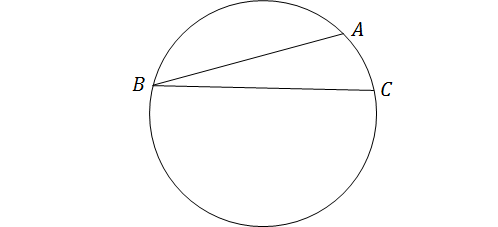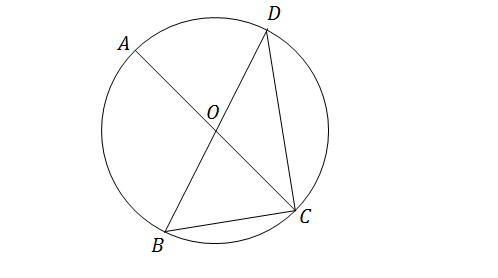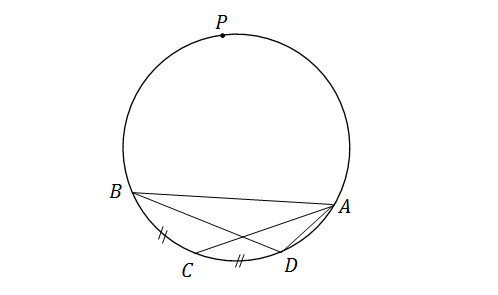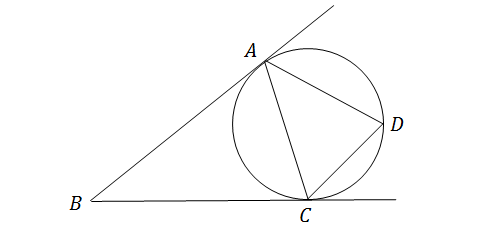Geometry

# Circles - Inscribed Angles

$A, B, C$ and $D$ are consecutive points on a circle (labeled clockwise) such that $\angle ABC = 73 ^\circ$. What is the measure of $\angle ADC$ (in degrees)?The above diagram shows a circle of radius $r,$ with $\angle ABC=15^\circ.$ If the length of arc $\widehat{AC}$ is equal to $10,$ what is the measure of $r?$

Note: The above figure is not drawn to scale.In the above diagram, point $O$ is the center of the circle. If $\angle BDC=26^\circ,$ what is $\angle BCA$ in degrees?

Note: The above figure is not drawn to scale.In the diagram above, the measures of the two arcs $\widehat{BC}$ and $\widehat{CD}$ are equal. If $\angle BAC=20^\circ$ and $\angle ABD=40^\circ,$ and the measure of arc $\widehat{BC}$ is equal to $6,$ what is the measure of arc $\widehat{APB}?$

Note: The above figure is not drawn to scale.In the diagram above, lines $AB$ and $BC$ are tangent lines of a circle, with points $A$ and $C$ as their respective tangent points. If $\angle ADC=70^\circ,$ what is $\angle ABC$ in degrees?

×